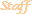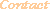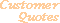Home Older Mathematica Items

Scientific Arts

# Stellar Structure and the Lane-Emden Function: Numerical Solution

Page 1 | Page 2

## Numerical Solution

### NDSolve

Here we want to numerically integrate Equation 1 and use the result to find the value of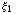. Chandrasekhar presents the following table, where the numerical results were obtained from a variety of sources.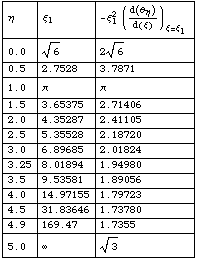Table 1

First try to use NDSolve for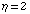.

``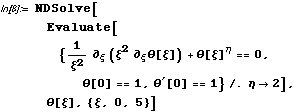``
`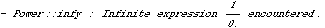`
``
`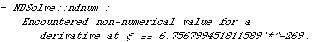`
`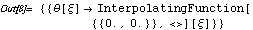`

Here the numerical integration was not able to leave the starting gate. The same problem happens when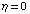(as well as in other cases).

``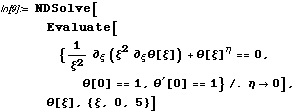``
``
``
``
`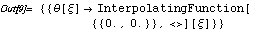`

But forthere is the simple analytic solution presented above.

The reason for the difficulty is seen in the expanded form of Equation 1.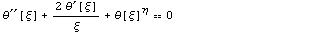From this we can see that, due to the potentially singular term, there might be difficulty with a numerical algorithm that starts at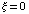. However, if we can move the boundary condition away from the origin, we can use NDSolve from that point on. We can use the differential equation, Equation 1, along with the boundary conditions at the origin, Equation 2, to make a series expansion for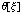about.

#### Series Expansion

We will create a general function for doing this, but will develop it by working with a particular case: a test case for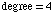.

``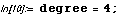``

First define the series in terms of unknown parameters.

``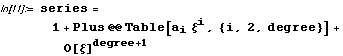``
`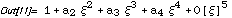`

In this expression the boundary conditions were imposed by choosing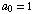and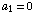. Now define a table of the unknown coefficients

``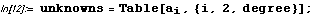``

The differential equation defines an expression for the unknown coefficients.

``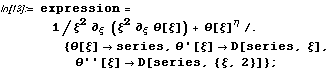``

Collect the coefficients in this expression.

``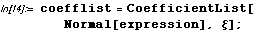``

Now solve the equations that result from setting the coefficients to zero (the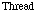in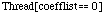creates the list of equations from the list of coefficients).

``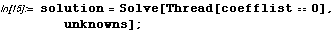``

Here is the resulting series.

``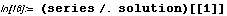``
`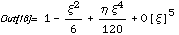`

Now we put all of this together into a function. We make the function self-documenting in the conventional Mathematica fashion through the device of a usage message.

``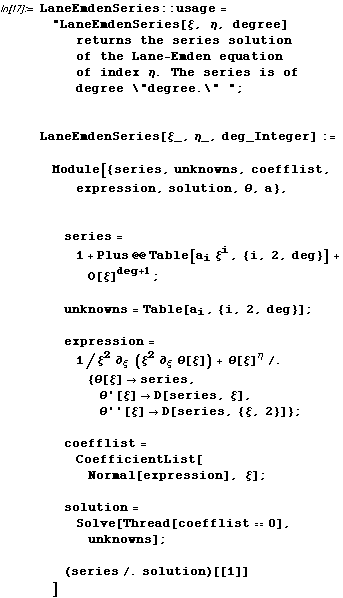``

The usage message allows us to access the definition of the function.

``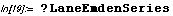``
`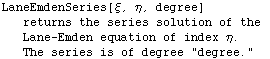`

It also enables the template creation feature the for the function (accessible through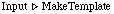menu item, or its keyboard equivalent).

Here is an example of the use of LaneEmdenSeries.

``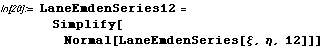``
`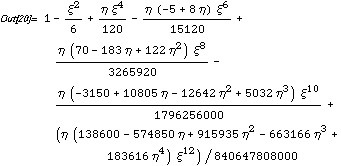`

(Chandrasekhar shows the result to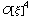; but, in his case we are sure the he could calculate it out to a significantly higher order: presumably he did. For mortals like us, we can do the same - without error - with Mathematica as a tool.)

#### Using the Series Solution in NDSolve

Since we designed the function LaneEmdenSeries to automatically incorporate the boundary conditions of Equation 2, it can be used to move the boundary condition to any other point away from the origin where the series is convergent. A prudent approach is to choose a point close to 0, say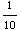, and explore the result.

First compute the new boundary conditions at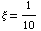.

``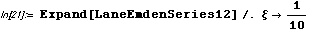``
`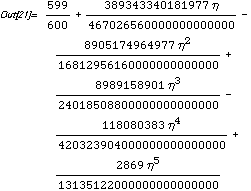`
````
`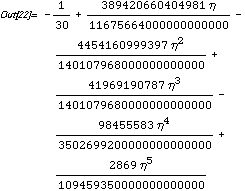`

Now attempt to use NDSolve with the new boundary conditions. We can experiment with machine arithmetic (the default) versus higher precision arithmetic by using various options to NDSolve. We find that the quoted results (of Table 1) are achieved in the latter case (for the series expansion point and degree that we have picked) if we set the option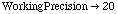.

Here is the result for(we use Module to localize the parameters).

``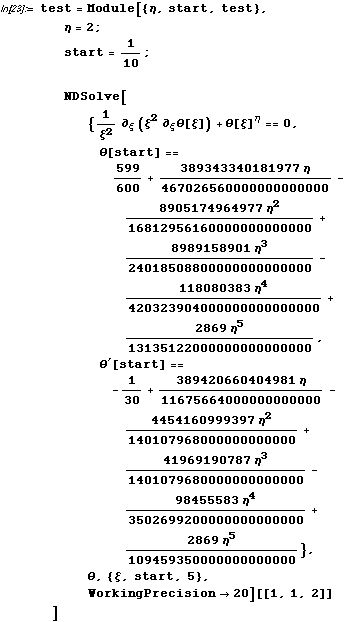``
`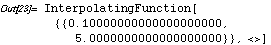`

Let's take a look at the result.

``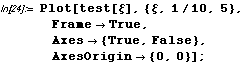``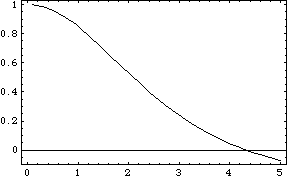Find the first real zero using FindRoot (with the option).

``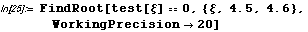``
`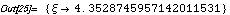`
``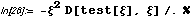``
`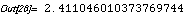`

These numbers agree with the results quoted in Table 1. The open question here is "how many of the digits of this answer are correct?" This can be investigated by changing the various options to NDSolve and FindRoot as well as the degree and starting point of the Lane-Emden series used to move the boundary conditions. The advantage of having access to arbitrary precision arithmetic is that you can investigate the numerical properties of a solution with much greater versatility than when you are under the constraints of machine arithmetic.

The procedure that we just used for the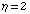case can be put together into a function so that you can experiment with and generate results for any case of interest. In this way you can reconstruct all of the entries of Table 1.

 For further information on our services send email to info@scientificarts.com . Contents of this web site Copyright © 1999-2011, Scientific Arts, LLC.
 s